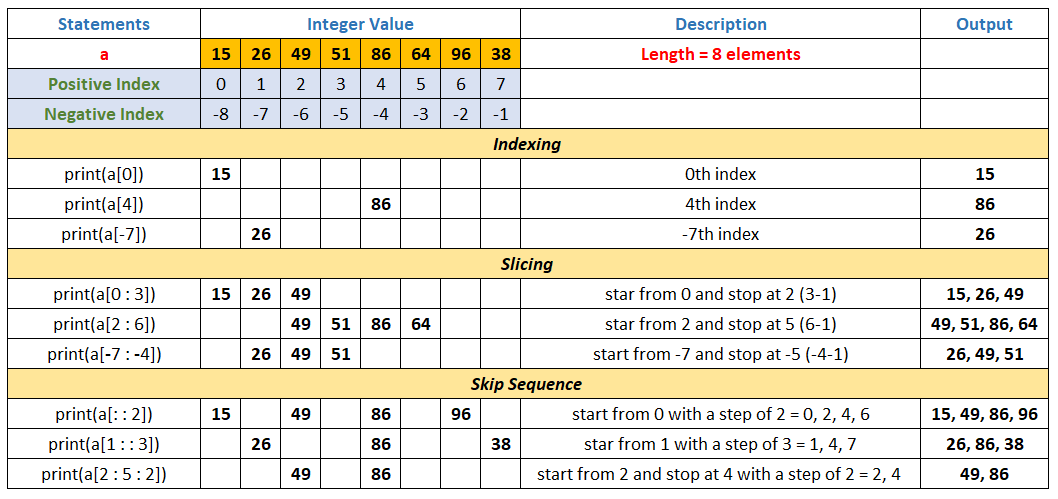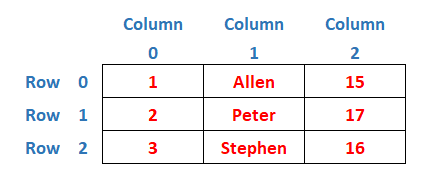# Tuples in Python Programming

###### Data Structures in Python

In this lesson, we will understand what is Tuple in Python Programming along with some examples.

## What is Tuple in Python?

A Tuple in Python is an immutable sequence. A Tuple is similar to a list, but one major difference between tuple and list is that a tuple is immutable but, a list is mutable. It means that we cannot change the element in tuple once it is defined.### Declaration Syntax of a Tuple in Python

``tuple_name = (elements1,element2,...)``

#### Example

``a = (5,8,6,2,9)     # A tuple with five elements in it``
Note: A tuple uses round brackets ( ) and a list uses square brackets [ ]

## Creating an Integer Type Tuple

A python program to show how to create and display an integer type tuple in python.

#### Example

``````a=(15,2,49,51,86)

# printing the elements from an integer type tuple
for x in a:
print(x)``````

```15
2
49
51
86```

## Creating a Floating Point Number Type Tuple

A python program to show how to create and display a floating point number type tuple in python.

#### Example

``````a=(18.23,24.11,564.2369,44.17,98.513)

# printing the elements from a floating point number type tuple
for x in a:
print(x)``````

```18.23
24.11
564.2369
44.17
98.513```

## Creating a Character Type Tuple

A python program to show how to create and display a character type tuple in python.

#### Example

``````a=('a','e','i','o','u')

# printing the elements from a character type tuple
for x in a:
print(x)``````

```a
e
i
o
u```

## Creating a String Type Tuple

A python program to show how to create and display a string type tuple in python.

#### Example

``````a=('apple','ball','cat','dog','eagle')

# printing the elements from a string type tuple
for x in a:
print(x)``````

```apple
ball
cat
dog
eagle```

## Creating a Tuple of Different Types of Elements

A python program to show how to create and display a tuple of different types of elements in python.

#### Example

``````a=(15,'Henry','2A',56,81,74,70.33,'B')

# printing the elements from a tuple having different types of elements
for x in a:
print(x)``````

```15
Henry
2A
56
81
74
70.33
B```

## Create Tuples from Existing Sequences

A python program to show how to create tuples from existing sequences. A sequence can be any kind of sequence including string, tuples and lists.

#### Example

``````str='Python'    # A String
name=['Allen','Peter','Henry','Stephen']    # A List
t=(12,89,63)    # A Tuple

T1=tuple(str)        # Creating a tuple from a String
T2=tuple(name)       # Creating a tuple from another List
T3=tuple(t)          # Creating a tuple from a Tuple

print(T1)
print(T2)
print(T3)``````

#### Output

```('P', 'y', 't', 'h', 'o', 'n')
('Allen', 'Peter', 'Henry', 'Stephen')
(12, 89, 63)```

## Tuple Slicing

Tuple slicing is the process of accessing a part of the tuple using either positive or negative indexing with the help of tuple slicing syntax given below.

``tuple_name[ start : end+1 : step]``#### Example

``````a=(15,26,49,51,86,64,96,38)

print(a)
print(a)
print(a[-7])
print(a[0:3])
print(a[2:6])
print(a[-7:-4])
print(a[::2])
print(a[1::3])
print(a[2:5:2])``````

```15
86
26
(15, 26, 49)
(49, 51, 86, 64)
(26, 49, 51)
(15, 49, 86, 96)
(26, 86, 38)
(49, 86)```

## Joining Tuples

We can use the concatenation operator + to join two tuples and returns the concatenated tuple as shown below.

#### Example

``````a=(15,2,49,51,86)
b=(56,8,97,4)
c=a+b

print(a)
print(b)
print(c)``````

#### Output

```(15, 2, 49, 51, 86)
(56, 8, 97, 4)
(15, 2, 49, 51, 86, 56, 8, 97, 4)```

## Use of Membership Operator in Tuple

Both in and not in operators works on Tuple just like they work for other sequences such as strings and lists. The in operator tells if an element is present in the tuple or not and not in does the opposite. See the example given below.

#### Example

``````a=(15,2,49,51,86)

print(a)
if (49 in a) == True:
print('49 is prenest in the tuple')
else:
print('49 is not prenest in the tuple')

if (70 not in a) == True:
print('70 is not prenest in the tuple')
else:
print('70 is prenest in the tuple')``````

#### Output

```(15, 2, 49, 51, 86)
49 is prenest in the tuple
70 is not prenest in the tuple```

## Tuple Methods

Tuple methods in Python are a collection of useful functions used to perform a specific task on a tuple. Let's see all the important tuple methods one by one with examples.

### count() Method

The count() method returns the number of occurrences of a specified element in an existing tuple.

#### Example

``````a=(11,9,26,34,26,84,97,26)
print('Original Tuple: ',a)
print('Total occurrence of element 26: ',a.count(26))``````

#### Output

```Original Tuple:  (11, 9, 26, 34, 26, 84, 97, 26)
Total occurrence of element 26:  3```

### index() Method

The index() method returns the index of the first occurrence of the specified element in an existing tuple. The index() method raise ValueError if the element is not found.

#### Example

``````a=(11,9,26,34,26,84,97,26)
print('Original Tuple: ',a)
print('Index of first occurrence of element 26: ',a.index(26))``````

#### Output

```Original Tuple:  (11, 9, 26, 34, 26, 84, 97, 26)
Index of first occurrence of element 26:  2```

## Functions to Process Tuples

There are some useful functions available in python that we can apply on a tuple. The difference between Function and Method is that a Function is called directly with its name but a Method is called on objects only.

Let's see the functions given below with examples.

### max() Function

The max() function returns the biggest element in a tuple.

#### Example

``````a=(11,91,57,34,26,97,63,18)
print('Tuple: ',a)
print('Biggest element is :',max(a))``````

#### Output

```Tuple:  (11, 91, 57, 34, 26, 97, 63, 18)
Biggest element is :  97```

### min() Function

The min() function returns the smallest element in a tuple.

#### Example

``````a=(11,9,57,34,5,97,63,10)
print('Tuple: ',a)
print('Smallest element is :',min(a))``````

#### Output

```Tuple:  (11, 9, 57, 34, 5, 97, 63, 10)
Smallest element is : 5```

### len() Function

The len() function returns the total numbers of elements present in a tuple.

#### Example

``````a=(11,91,57,34,26,97,63,18)
print('Tuple: ',a)
print('Total number of elements :',len(a))``````

#### Output

```Tuple:  (11, 91, 57, 34, 26, 97, 63, 18)
Total number of elements : 8```

## Iterate all Elements in a Tuple using range() Function

We can also iterate all elements in a tuple using range() function as shown below.

#### Example

``````a=(15,2,49,51,86)

# printing the elements from an integer type tuple
for i in range(0,len(a)):
print(a[i])``````

```15
2
49
51
86```

## Nested Tuple

A tuple within another tuple is known as Nested Tuple. We can use nested tuple to create matrix in python. See the examples of nested tuple given below.

#### Example

A python program to show how to create a nested tuple to store the roll and names and age of three candidates.

``````a=((1,'Allen',15),(2,'Peter',17),(3,'Stephen',16))

# printing the entire tuple
print(a,'\n')

# printing the tuple row by row
for r in range(3):
for c in range(3):
print(a[r][c],end=' ')
print()``````

#### Output

```((1, 'Allen', 15), (2, 'Peter', 17), (3, 'Stephen', 16))

1 Allen 15
2 Peter 17
3 Stephen 16```

We can assume the above nested tuple as shown in the image below.We can access any cell of a nested tuple using the following syntax.

``tuple_name = [row_index][column_index]``

In the above program, we have run a nested for loop to access all the elements of the nested tuple. When the value of outer for loop r is 0, then the inner for loop c runs from 0 to 2 and prints all the elements of the first row of the nested tuple.

When the value of outer for loop r is 1, then again the inner for loop c runs from 0 to 2 and prints all the elements of the second row of the nested tuple and in the same way it prints the third row also.